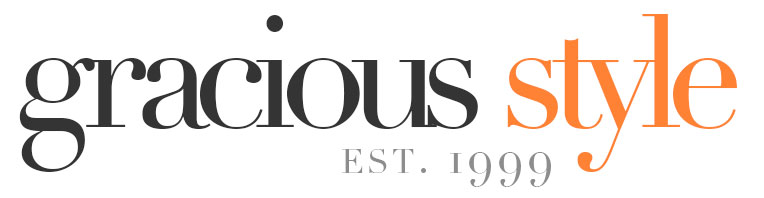## What Size Tablecloth Do I Need?

We get asked this question all the time, so we’ve put together this really simple guide.

1) First, measure the length and width of your table.  If you have a round table, just measure all the way across the top.

For example, let’s say your table is 48 in x 106 in.

2) Multiply the drop you’d like by two and add this to each of your dimensions.

The longer the drop, the more formal the look.  At home, we prefer a 10 inch drop because it looks elegant and keeps it from having too much fabric on our laps.  For special events like weddings, we always go to the floor.

- For standard formal dining, go with a 10 inch drop (add 20 inches to each dimension).

- For a lavish look, go with a 30 inch drop (add 60 inches to each dimension).

In our example, let’s go with a standard drop, so add 20 inches to each dimension: now you have 68 in x 126 in.

3) Find the tablecloth size that is closest to the numbers you just added.

In this case, the closest size is 70 in x 124 in.

4) Determine which shape you need: rectangular, round, square, or oval.

Following is a basic chart of standard tablecloth sizes and the table sizes they would fit: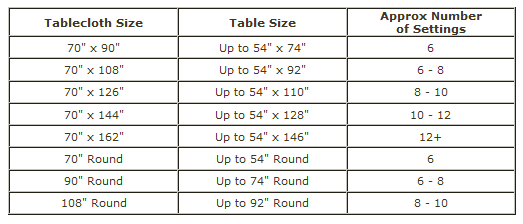Rectangular tablecloths (also called oblongs) fit both rectangular and oval tables.

Here’s a rectangular tablecloth on a rectangular table: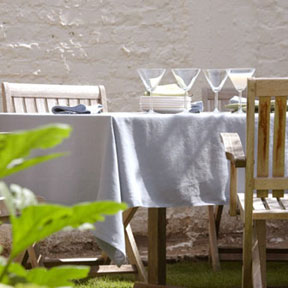Here’s a rectangular tablecloth on an oval table: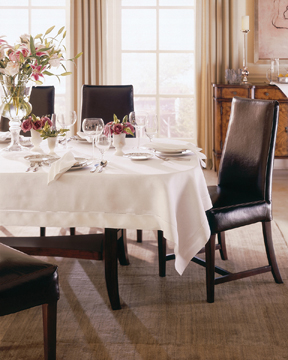Rounds tablecloths fit round tables.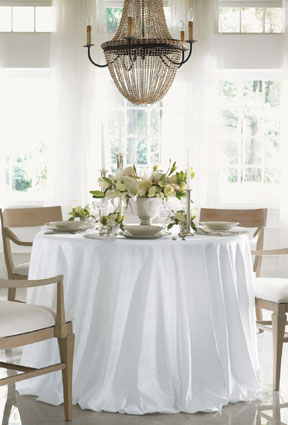Square tablecloths fit both square or round tables.

Here’s a square tablecloth on a square table: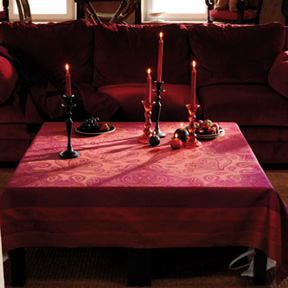Here’s a square tablecloth on a round table:And finally, oval tablecloths (also called racetrack ovals) fit only oval tables.